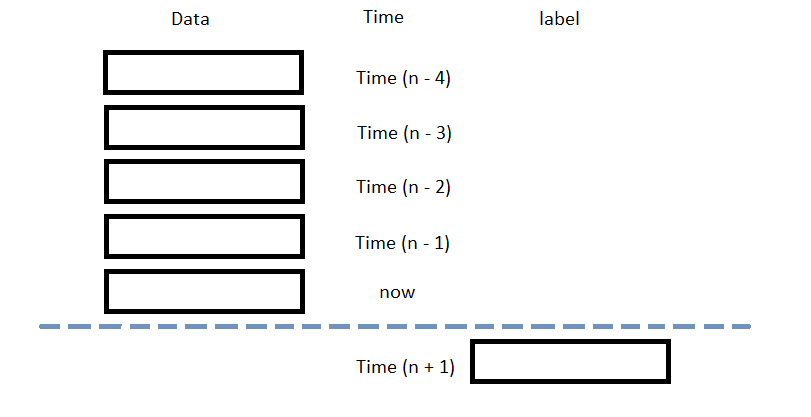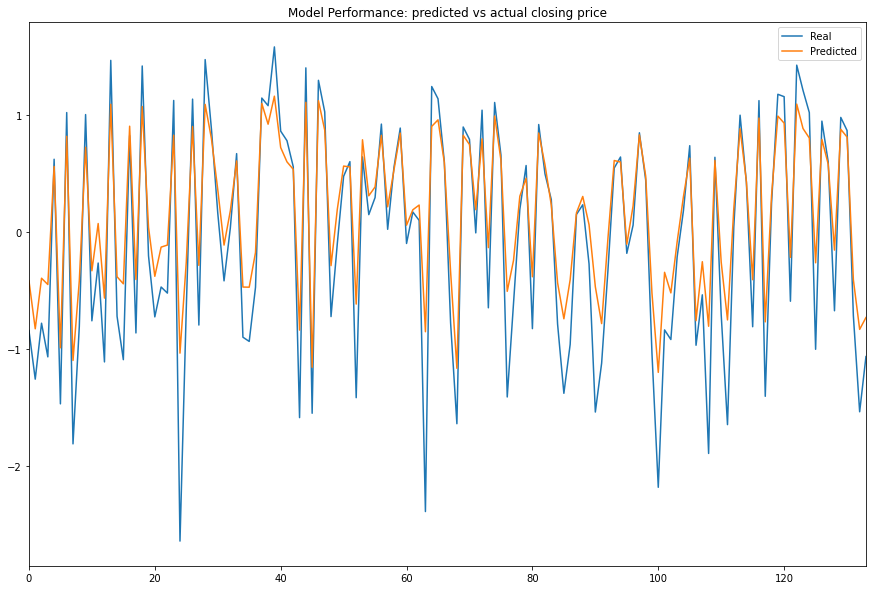# Machine Learning

## XGBoost

### Introduction

This page explains how to build, train, test, and store XGBoost models.

### Import Libraries

Import the xgboost, sklearn, and joblib libraries.

import xgboost as xgb
from sklearn.model_selection import train_test_split
import joblib

You need the sklearn library to prepare the data and the joblib library to save models.

### Get Historical Data

Get some historical market data to train and test the model. For example, to get data for the SPY ETF during 2020 and 2021, run:

qb = QuantBook()
history = qb.History(symbol, datetime(2020, 1, 1), datetime(2022, 1, 1)).loc[symbol]

### Prepare Data

You need some historical data to prepare the data for the model. If you have historical data, manipulate it to train and test the model. In this example, use the following features and labels:

Data CategoryDescription
FeaturesThe last 5 closing prices
LabelsThe following day's closing price

The following image shows the time difference between the features and labels:Follow these steps to prepare the data:

1. Perform fractional differencing on the historical data.
2. df = (history['close'] * 0.5 + history['close'].diff() * 0.5)[1:]

Fractional differencing helps make the data stationary yet retains the variance information.

3. Loop through the df DataFrame and collect the features and labels.
4. n_steps = 5
features = []
labels = []
for i in range(len(df)-n_steps):
features.append(df.iloc[i:i+n_steps].values)
labels.append(df.iloc[i+n_steps])
5. Convert the lists of features and labels into numpy arrays.
6. features = np.array(features)
labels = np.array(labels)
7. Standardize the features and labels
8. X = (features - features.mean()) / features.std()
y = (labels - labels.mean()) / labels.std()
9. Split the data into training and testing periods.
10. X_train, X_test, y_train, y_test = train_test_split(X, y)

### Train Models

We're about to train a gradient-boosted random forest for future price prediction.

1. Split the data for training and testing to evaluate our model.
2. X_train, X_test, y_train, y_test = train_test_split(X, y)
3. Format training set into XGBoost matrix.
4. dtrain = xgb.DMatrix(X_train, label=y_train)
5. Train the model with parameters.
6. params = {
'booster': 'gbtree',
'colsample_bynode': 0.8,
'learning_rate': 0.1,
'lambda': 0.1,
'max_depth': 5,
'num_parallel_tree': 100,
'objective': 'reg:squarederror',
'subsample': 0.8,
}
model = xgb.train(params, dtrain, num_boost_round=10)

### Test Models

We then make predictions on the testing data set. We compare our Predicted Values with the Expected Values by plotting both to see if our Model has predictive power.

1. Format testing set into XGBoost matrix.
2. dtest = xgb.DMatrix(X_test, label=y_test)
3. Predict with the testing set data.
4. y_predict = model.predict(dtest)
5. Plot the result.
6. df = pd.DataFrame({'Real': y_test.flatten(), 'Predicted': y_predict.flatten()})
df.plot(title='Model Performance: predicted vs actual closing price', figsize=(15, 10))
plt.show()### Store Models

#### Saving the Model

We dump the model using the joblib module and save it to Object Store file path. This way, the model doesn't need to be retrained, saving time and computational resources.

1. Set the key name of the model to be stored in the Object Store.
2. model_key = "model"
3. Call GetFilePath with the key's name to get the file path.
4. file_name = qb.ObjectStore.GetFilePath(model_key)
5. Call dump with the model and file path to save the model to the file path.
6. joblib.dump(model, file_name)

Let's retrieve the model from the Object Store file path and load by joblib.

1. Call the ContainsKey method.
2. qb.ObjectStore.ContainsKey(model_key)

This method returns a boolean that represents if the model_key is in the Object Store. If the Object Store does not contain the model_key, save the model using the model_key before you proceed.

3. Call GetFilePath with the key's name to get the file path.
4. file_name = qb.ObjectStore.GetFilePath(model_key)
5. Call load with the file path to fetch the saved model.
6. loaded_model = joblib.load(file_name)

y_pred = loaded_model.predict(dtest)
df.plot(title='Model Performance: predicted vs actual closing price', figsize=(15, 10))